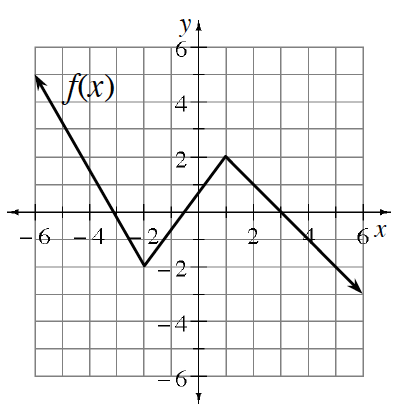### Home > INT1 > Chapter 10 > Lesson 10.2.2 > Problem10-91

10-91.

Given the graph of $f\left(x\right)$ right, graph each transformation listed below.

1. $f(x) + 1$

1. $f(x) − 2$